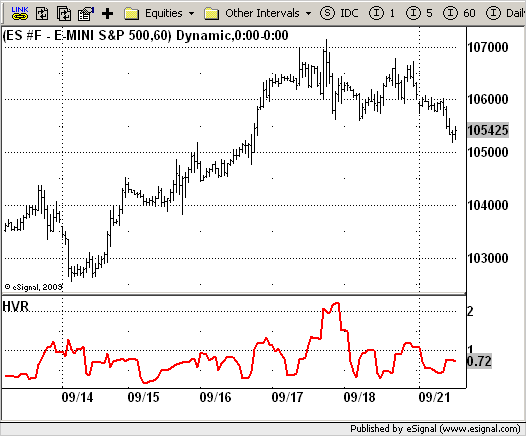# Historical Volatility Ratio

ICE Data Services -

File Name: HVR.efs

Description:
Historical Volatility Ratio

Formula Parameters:
FastLength : 6
SlowLength : 100

Notes:
The HVR is basically a mathematical ratio or percentage of a short
to a long average historical volatility. When a market's short volatility
declines below a certain percentage of its long volatility, it is a heads
up signal that an explosive move may be imminent.
The standard settings used are both the 10/100 and 6/100 HVR with a 50% ratio.
The 50% ratio will be the trigger point. If either the 10/100 OR the 6/100
declines below 50% in any market, that market should be watched for potential
trades, as a sharp move could be seen.
Both the 10/100 and the 6/100 HVR indicators are included in this package.

HVR.efsEFS Code:

```/*********************************
Provided By:
eSignal (Copyright c eSignal), a division of Interactive Data
Formula Script (EFS) is for educational purposes only and may be
modified and saved under a new file name.  eSignal is not responsible
for the functionality once modified.  eSignal reserves the right
to modify and overwrite this EFS file with each new release.

Description:
Historical Volatility Ratio

Version:            1.0  09/30/2009

Formula Parameters:                     Default:
FastLength                          6
SlowLength                          100

Notes:
The HVR is basically a mathematical ratio or percentage of a short
to a long average historical volatility. When a market's short volatility
declines below a certain percentage of its long volatility, it is a heads
up signal that an explosive move may be imminent.
The standard settings used are both the 10/100 and 6/100 HVR with a 50% ratio.
The 50% ratio will be the trigger point. If either the 10/100 OR the 6/100
declines below 50% in any market, that market should be watched for potential
trades, as a sharp move could be seen.
Both the 10/100 and the 6/100 HVR indicators are included in this package.

**********************************/
var fpArray = new Array();
var bInit = false;

function preMain(){
setPriceStudy(false);
setShowCursorLabel(true);
setShowTitleParameters(false);
setStudyTitle("HVR");
setCursorLabelName("HVR", 0);
setPlotType(PLOTTYPE_LINE, 0);
setDefaultBarFgColor(Color.red, 0);
setDefaultBarThickness(2,0);
var x = 0;
fpArray[x] = new FunctionParameter("FastLength", FunctionParameter.NUMBER);
with(fpArray[x++]) {
setLowerLimit(1);
setDefault(6);
}
fpArray[x] = new FunctionParameter("SlowLength", FunctionParameter.NUMBER);
with(fpArray[x++]) {
setLowerLimit(1);
setDefault(100);
}
}

var xCloseLog = null;
var xCLMA6 = null;
var xCLMA100 = null;

function main(FastLength, SlowLength) {
var nBarState = getBarState();
var nRes = 0;
var hv6 = 0;
var hv100 = 0;
var i = 0;
if (nBarState == BARSTATE_ALLBARS) {
if(FastLength == null) FastLength = 6;
if(SlowLength == null) SlowLength = 100;
}
if (bInit == false) {
xCloseLog = efsInternal("Calc_CloseLog");
xCLMA6 = sma(FastLength, xCloseLog);
xCLMA100 = sma(SlowLength, xCloseLog);
bInit = true;
}
if (xCloseLog.getValue(-SlowLength) == null) return;
for(i =0; i < FastLength; i++) {
hv6 = hv6 + Math.pow(xCloseLog.getValue(-i) - xCLMA6.getValue(0), 2);
}
hv6 = Math.sqrt(hv6 / FastLength) * 7.2111;
for(i=0; i < SlowLength; i++) {
hv100=hv100 + Math.pow(xCloseLog.getValue(-i) - xCLMA100.getValue(0), 2);
}
hv100 = Math.sqrt(hv100 / SlowLength) * 7.2111;
nRes = hv6/hv100;
return nRes;
}

function Calc_CloseLog() {
var nRes = 0;
nRes =  Math.log(close(0) / close(-1));
return nRes;
}
```